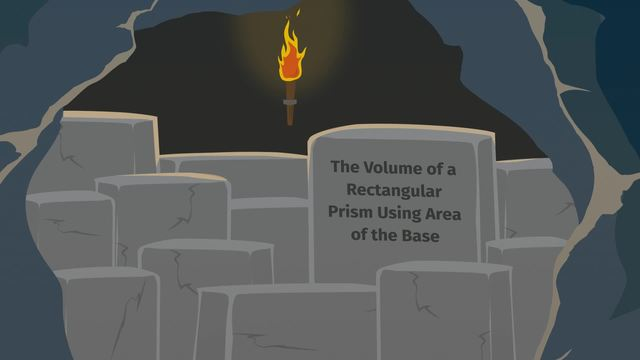# The Volume of a Rectangular Prism Using the Area of the BaseRating

Ø 5.0 / 1 ratings
The authorsChris S.

## Basics on the topicThe Volume of a Rectangular Prism Using the Area of the Base

After this lesson you will be able to develop and use the formula V=Bh.

The lesson begins with the formula for a rectangular prism, V=lwh. It leads to calculating the volume of rectangular prisms with the same area of base and different heights. It concludes with a new formula for finding the volume of a rectangular prism, V=Bh, where B is the area of the base.

Learn how to use the area of base to find the volume of a rectangular prism by helping Rocky fit all of his stones in storage!

This video includes key concepts, notation, and vocabulary such as: area of base (the base of a rectangular prism is an arbitrarily chosen rectangular face); height (the height is the remaining dimension after we have calculated the area of the base); and volume of a rectangular prism (Volume equals the area of the base times the height, V=Bh).

Before watching this video, you should already be familiar with fractions, calculating area for rectangles, and calculating volume by filling with cubes or using the formula, V=lwh.

After watching this video, you will be prepared to learn that volume changes when we double or triple the dimensions of the prism.

Common Core Standard(s) in focus: 6.G.A.2 A video intended for math students in the 6th grade Recommended for students who are 11-12 years old

### TranscriptThe Volume of a Rectangular Prism Using the Area of the Base

Gem is reading the latest issue of Better Caves and Firepits magazine. Stone blocks used to be popular decoration, but the world has moved on! Unless they want to be cultural dinosaurs, Gem's husband Rocky must remove his stone collection. Rocky is attached to his stones but he has an idea! He can try to fit all his stones in his storage cave. If Rocky can calculate the volume of a rectangular prism using the area of the base he just might fit all his stone blocks in storage. Rocky has 3 different sizes of stone blocks. How can Rocky calculate the volume of each? The formula for the volume of a rectangular prism is length times width times height. The volume of the first block is the length, 5 times the width, 2 times the height which is also 2. That's 10 times 2 or 20 cubic inches. The volume of the second is 5 times 2 times 7. That's 10 times 7 or 70 cubic inches. The volume of the third is 5 times 2 times 11. That's 10 times 11 or 110 cubic inches. Notice that each prism has the same length and width. In each case, we first multiplied 5 times 2 to get 10. This leads to another way of thinking about the volume of a rectangular prism. Each prism is composed of 6 rectangular faces. The base of each prism is the face on the bottom. And so the height is the side length perpendicular to the base. The area of the base.. of each block is 5 times 2 or 10 square inches. To find the total volume, we multiply 10 times the height of each prism. In general, we can calculate the volume of a prism by finding the area of the base times the height. We can use this equation anytime we are calculating the volume of a prism. Rocky tries to neatly fit his beloved blocks into his storage cave. But some of the blocks will need to be flipped on their sides so they can fit properly. Rocky is a bit concerned--will the volumes still be the same if a different face is on the bottom? Let's take the 5 by 2 by 7 block as an example. With the 5 by 2 face as the base the area of the base is 10 the volume is the base times the height, 7 giving us a volume of 70 cubic inches. Let's flip the block on its side. Now, the seven by 2 face is on the bottom. It's the new base of the prism, and the area of the base is 7 times 2 or 14 square inches. Multiplying 14 times the new height of 5 gives us a volume of 70 cubic inches, as before. Let's flip the block one more time so that the 7 by five face is the base. The area of the base is 7 times 5 or 35 square inches. Multiplying 35 times the new height of 2 confirms the volume of 70 cubic inches. With a rectangular prism, any face can be the base and the volume can be computed by calculating the area of the base times the height. Rocky can flip his blocks freely without changing the volume--that makes his work easier! Let's review our tools for calculating the volume of a rectangular prism. We can calculate the volume as length times width times height. We can also select any face of a rectangular prism as the base. We then calculate the area of the base, and multiply by the height. Rocky has efficiently stored all his stones, and Gem is very grateful. She tells him that he can keep his stones in storage there for as long as he likes. Mmm if only they knew it was just an ordinary caveman cleaning his cave.